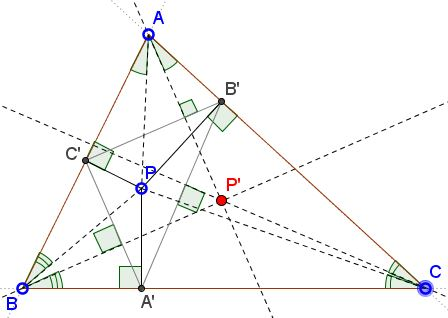# Pedal Triangle and Isogonal ConjugacyWhat is this about? A Mathematical Droodle

Explanation### Pedal Triangle and Isogonal Conjugacy

The applet intends to suggest the following results:

Let P be a point in the plane of ΔABC.Then:

1. The pedal triangle A'B'C' of P is orthologic to ΔABC. In particular, the perpendiculars from the vertices of A, B, C to the opposite sides of ΔA'B'C' meet in a point, say, P'.

2. P' is the isogonal conjugate of P.

### Proof

The first assertion is an immediate consequence of the definition of the pedal triangle: the vertices of the pedal triangle of point P are the feet of perpendiculars from P to the sides of ΔABC. Naturally then the perpendiculars from P to the sides of ΔABC are concurrent: they meet in P. By Maxwell's theorem, the perpendiculars from the vertices of ΔABC onto the sides of ΔA'B'C' are also concurrent.

To see that point P' of concurrency is the isogonal conjugate of P, observe that in quadrilateral AB'PC' the angles at B' and C' are right. The quadrilateral is therefore cyclic. Which implies that angles APC' and AB'C' are equal (as inscribed angles subtending the same arc.) Let X be the foot of perpendicular from A to B'C' (that passes through P'.) It follows that the right triangles APC' and AB'X are similar and their other acute angles are also equal:

∠C'AP = ∠XAB' = ∠P'AB',

so that AP and AP' are isogonal to each other. The same consideration applies to the vertices B and C, thus proving the second assertion.

(The first property is mentioned in [Honsberger, p. 64]. Both properties are offered as exercises in [TCCT, p. 215].)

### References

1. R. Honsberger, Episodes in Nineteenth and Twentieth Century Euclidean Geometry, MAA, 1995, pp. 153-154
2. C. Kimberling, Triangle Centers and Central Triangles, Congressus Numerantium 129, Utilitas Mathematica Publishing, 1998.### Maxwell Theorem and orthologic triangles